Courses

# Equimolar Counter Diffusion Of A and B (NA =-NB) Chemical Engineering Notes | EduRev

## Chemical Engineering : Equimolar Counter Diffusion Of A and B (NA =-NB) Chemical Engineering Notes | EduRev

The document Equimolar Counter Diffusion Of A and B (NA =-NB) Chemical Engineering Notes | EduRev is a part of the Chemical Engineering Course Mass Transfer.
All you need of Chemical Engineering at this link: Chemical Engineering

Equimolar counter-diffusion of A and B (NA =-NB)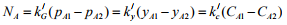→ Gas phase                (3.19)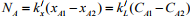→ Liquid phase                                        (3.20)

For gas phase diffusion we know,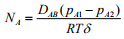(3.21)

Equating Equation (3.19) and Equation (3.21),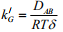(3.22)

Again,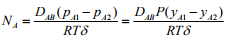(3.23)

Equating Equation (3.19) and Equation (3.23),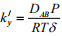(3.24)
Also,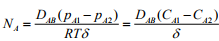(3.25)
Equating Equation (3.19) and Equation (3.25),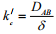(3.26)

For liquid phase diffusion the flux can be written as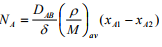(3.27)
Equating Equation (3.20) and Equation (3.27),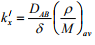(3.28)
Also,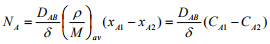(3.29)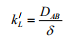(3.30)

Equating Equation (3.8) and Equation (3.17),
Conversions: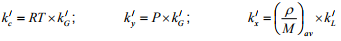Example problem 3.1:
Hydrochloric acid (A) diffuses through a thin film of water (B) 4.0 mm thick at 283 K. The concentration of HCl at point 1 on one boundary of the film is 12 wt% and on the other boundary, at point 2 is 4 wt%. The diffusivity of HCl in water is 2.5 x 10-9 m2/s. Calculate the flux of HCl considering water to be stagnant. Density of the solutions at points 1 and 2 are 1060.7 kg/m3 and 1020.15 kg/m3 respectively.

Solution 3.1: Molecular weight of HCl, MA = 36.5 and MB = 18
At point 1, mole fraction of HCl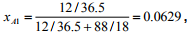Therefore, xB1 = 1- 0.0629 = 0.9371
Average molecular weight at point 1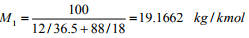At point 2, mole fraction of HCl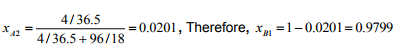Average molecular weight at point 2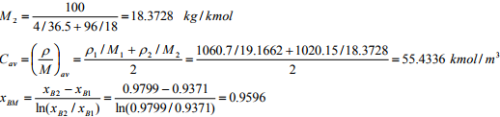z = 4 mm = 0.004 m
Flux of HCl,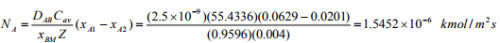3.2 Mass transfer under laminar flow condition
Mass transfer coefficient does not play a big role in laminar flow condition as molecular diffusion exists there. In laminar flow regime, the average liquid phase mass transfer coefficient, kL,av is correlated with Sherwood number (Sh) and DAB as follows: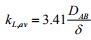(3.31)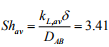(3.32)

3.3 Mass transfer under turbulent flow past solids
Mass transfer under flow past solid is a practically useful situation. Several theories have attempted to clarify the behavior of mass transfer coefficients. All the theories have some assumptions and some drawbacks. Hence, these are revised frequently. In turbulent flow medium, small fluid an element of different sizes, called eddies, move randomly. These eddies form, interact among others and disappear in the flow path. The total molar flux of a solute ‘A’ due to molecular diffusion and eddy diffusion, JA is as follows: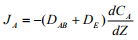(3.33)
where DE is eddy diffusivity. Eddy diffusivity depends on intensity of local turbulence.

Offer running on EduRev: Apply code STAYHOME200 to get INR 200 off on our premium plan EduRev Infinity!

## Mass Transfer

29 videos|46 docs|44 tests

,

,

,

,

,

,

,

,

,

,

,

,

,

,

,

,

,

,

,

,

,

;